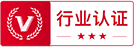QQ群反馈# 【新像素】详解AE常用的表达式

605 35 15 1
2020-06-19
7.3AE里可以打关键帧的属性前面都会有码表，我们按着Alt键+鼠标左键点击码表，就可以调出来属性的表达式输入框，接下来在里面输入想要添加的表达式就可以了。1、time时间表达式

time表达式的写法是time*n，n表示单位时间(每秒)内，属性参数的变化量。如给图层的旋转属性添加表达式：time*90，则表示该图层每秒钟旋转90度，并且旋转会一直持续下去。2、wiggle抖动表达式

wiggle是很常用的表达式，对物体实现随机抖动。写法是wiggle(x,y)，x表示每秒钟抖动的频率，y表示抖动的幅度，即每次抖动所偏移的量。3、loopOut循环表达式

loopOut表达式可以创建循环的动画效果，我们只需要做动画的开始帧、结束帧，添加表达式后动画就会一直循环运动，不用再重复打关键帧了。loopOut(type = "cycle", numKeyframes = 0)loopOut循环表达式的类型，最常用的是“cycle”，指的是在动画的最后一个关键帧结束，然后从第一个关键帧开始循环播放。4、惯性弹跳表达式e = .7;

g = 5000;

nMax = 9;

n = 0;

if (numKeys > 0){

n = nearestKey(time).index;

if (key(n).time > time) n--;

}

if (n > 0){

t = time - key(n).time;

v = -velocityAtTime(key(n).time - .001)*e;

vl = length(v);

if (value instanceof Array){

vu = (vl > 0) ? normalize(v) : [0,0,0];

}else{

vu = (v < 0) ? -1 : 1;

}

tCur = 0;

segDur = 2*vl/g;

tNext = segDur;

nb = 1; // number of bounces

while (tNext < t && nb <= nMax){

vl *= e;

segDur *= e;

tCur = tNext;

tNext += segDur;

nb++

}

if(nb <= nMax){

delta = t - tCur;

value +  vu*delta*(vl - g*delta/2);

}else{

value

}

}else

value5、random随机表达式

random表达式，可以实现随机变化的效果。写法是random(min,max)，min表示最小值，max表示最大值，添加表达式后会在最小值与最大值之间随机取数值进行变化（很多人容易把random与wiggle搞混，wiggle是控制两个不同属性参数的变化）。6、index图层编号表达式

index指的是AE图层前面的编号，使用这个表达式可以非常便捷的做出有规律的变化效果。7、延迟表达式opacityFactor = .75;

Math.pow(opacityFactor,index - 1)*1008、弹性表达式

amp = .1;

freq = 2.0;

decay = 2.0;

n = 0;

if (numKeys > 0){

n = nearestKey(time).index;

if (key(n).time > time){n--;}

}

if (n == 0){ t = 0;}

else{t = time - key(n).time;}

if (n > 0){

v = velocityAtTime(key(n).time - thisComp.frameDuration/10);

value + v*amp*Math.sin(freq*t*2*Math.PI)/Math.exp(decay*t);

}

else{value}# 新像素教育

2019全日制UI训练营名额预约中 官网：uixxs.com

2372粉丝/7关注1##### 浪pi6-20 11:17

2019全日制UI训练营名额预约中 官网：uixxs.com0/200

• 全部设计师
• 推荐设计师和认证设计师

### 您确认要推荐？

#### 推荐心得

0/200//